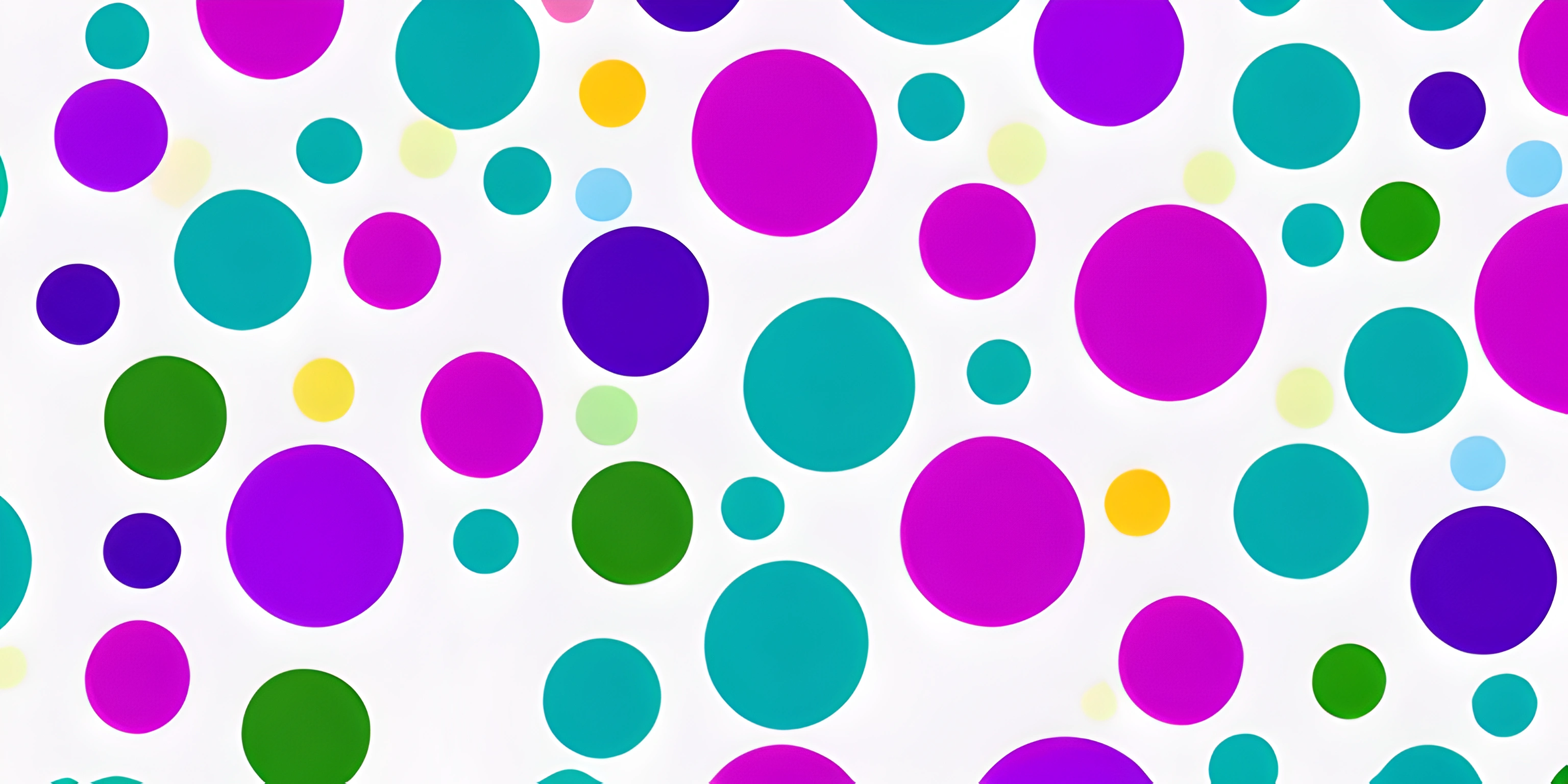# Graphs BasicsNote: this page has been created with the use of AI. Please take caution, and note that the content of this page does not necessarily reflect the opinion of Cratecode.

Graphs are everywhere! No, we're not talking about those bar and pie charts. We're diving into the wonderful world of graph data structures, a versatile and powerful way to represent relationships between objects. So buckle up and get ready to explore the basics of graphs and their uses.

## What is a Graph?

In computer science, a graph is a data structure that consists of a finite set of nodes (also known as vertices) and a set of edges connecting these nodes. Each edge represents a relationship between two nodes. Graphs are incredibly useful for modeling and solving problems that involve connections or relationships between objects, such as social networks, transportation networks, or even the internet itself!

There are two main types of graphs: directed and undirected. In directed graphs, edges have a direction, meaning that the relationship between the nodes has a specific order. In undirected graphs, edges have no direction, and the relationship between nodes is mutual.

Let's take a look at a simple example of an undirected graph:

``````A -- B
|    |
|    |
C -- D``````

In this graph, there are four nodes (A, B, C, and D) and four edges connecting them. Notice that there are no arrows or directions associated with the edges.

## Basic Terminology

Before we continue, let's get familiar with some basic graph terminology:

1. Node (or Vertex): The fundamental unit of a graph, representing an individual object, entity, or data point.
2. Edge: A connection or relationship between two nodes in the graph.
3. Degree: The number of edges connected to a node. In a directed graph, we have two types of degrees:
• In-degree: The number of incoming edges to a node.
• Out-degree: The number of outgoing edges from a node.
4. Path: A sequence of nodes and edges connecting two nodes in the graph.
5. Cycle: A path that starts and ends at the same node, without repeating any other nodes or edges.
6. Adjacency: Two nodes are adjacent if there is a direct edge between them.

## Why Use Graphs?

Graphs are incredibly versatile and can be used to solve a wide range of problems, such as:

1. Social Network Analysis: Graphs can be used to model and analyze relationships between people, organizations, or groups within a social network.
2. Pathfinding and Route Optimization: Graphs are widely used in mapping and navigation applications to find the shortest or most efficient route between two locations.
3. Dependency Analysis: In software development, graphs can be used to model dependencies between components or modules, helping to identify potential issues and optimize the overall architecture.
4. Web Crawling and Search: Graphs play a crucial role in modeling the structure of the web and enable search engines to efficiently crawl, index, and rank websites based on their connectivity and relevance.

These are just a few examples of the many applications of graphs in computer science and beyond. By understanding the basics of graph data structures, you'll be well-equipped to tackle a wide range of problems and make connections that were previously unimaginable.

Now that you've been introduced to the world of graphs, feel free to explore more advanced concepts such as graph traversal algorithms, weighted graphs, and graph optimization problems.